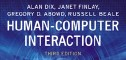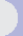HCI book > exercises > 17. system models > ex. 17.3 text onlyexercises17. models of the systemEXERCISE 17.3

In Section 17.2.5, we said that the specification Algebraic-draw could be extended to say that a move, resize or unselect after a delete has no effect. The axiom for unselect looks like this:

(9) unselect(delete(g)) = delete(g)

Write two more axioms (10) and (11) which say the same about move and resize. Now use axioms (4) and (5) to show that (9) implies both your new axioms.

(9) unselect(delete(st)) = delete(st)

(10) move(p,delete(st)) = delete(st)

(11) resize(p,delete(st)) = delete(st)

Axioms 4 and 5 are:

(4) move(p,unselect(st)) = unselect(g)

(5) resize(p,unselect(st)) = unselect(g)

To show that Axiom 10 is derivable from Axioms 4 and 9 we can argue as follows:

 move(p,delete(st)) = move(p,unselect(delete(st)) by Axiom 9 = unselect(delete(st)) by Axiom 4 = delete(st) by Axiom 9

A similar argument shows that Axiom 11 is derivable from Axioms 5 and 9.

 Other exercises in this chapter ex.17.1 (ans), ex.17.2 (ans), ex.17.3 (ans), ex.17.4 (ans), ex.17.5 (tut), ex.17.6 (tut), ex.17.7 (tut), ex.17.8 (tut), ex.17.9 (tut)all exercises for this chapter